### Create an Account

Home / Questions / In Problem 428 determine the magnitude of the velocity of A and B when they have moved 500...

# In Problem 428 determine the magnitude of the velocity of A and B when they have moved 500 mm if the coefficient of kinetic friction between all surfaces is Strategy The simplest approach is to

In Problem 4.28, determine the magnitude of the velocity of A and B when they have moved 500 mm if the coefficient of kinetic friction between all surfaces is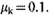Strategy: The simplest approach is to apply the principle of work and energy to A and B individually. If you treat them as a single system, you must account for the work done by internal friction forces. Sec Example 4.3.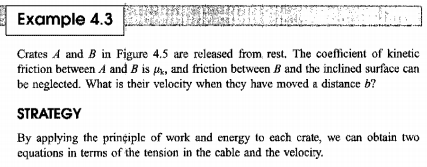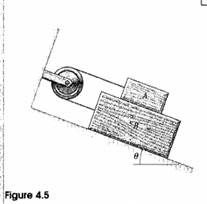Problem 4.28

The masses of the three blocks are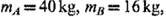and me = 12 kg. Neglect the mass of the bar holding C in place. Friction is negligible, By applying the principle of work and energy to A and B individually, determine the magnitude of their velocity when they have moved 500 rom.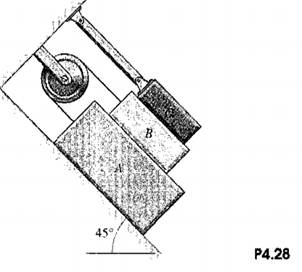Jun 16 2020 View more View LessSubscribe To Get Solution